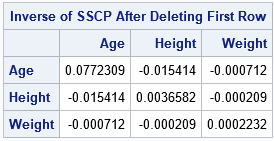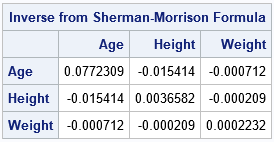For linear regression models, there is a class of statistics that I call deletion diagnostics or leave-one-out statistics. These observation-wise statistics address the question, "If I delete the i_th observation and refit the model, what happens to the statistics for the model?" For example:

• The PRESS statistic is similar to the residual sum of squares statistic but is based on fitting n different models, where n is the sample size and the i_th model excludes the i_th observation.
• Cook's D statistic measures the influence of the i_th observation on the fit.
• The DFBETAS statistics measure how the regression estimates change if you delete the i_th observation.

Although most references define these statistics in terms of deleting an observation and refitting the model, you can use a mathematical trick to compute the statistics without ever refitting the model! For example, the Wikipedia page on the PRESS statistic states, "each observation in turn is removed and the model is refitted using the remaining observations. The out-of-sample predicted value is calculated for the omitted observation in each case, and the PRESS statistic is calculated as the sum of the squares of all the resulting prediction errors." Although this paragraph is conceptually correct, theSAS/STAT documentation for PROC GLMSELECT states that the PRESS statistic "can be efficiently obtained without refitting the model n times."

### A rank-1 update to the inverse of a matrix

Recall that you can use the "normal equations" to obtain the least squares estimate for the regression problem with design matrix X and observed responses Y. The normal equations are b = (X`X)-1(X`Y), where X`X is known as the sum of squares and crossproducts (SSCP) matrix and b is the least squares estimate of the regression coefficients. For data sets with many observations (very large n), the process of reading the data and forming the SSCP is a relatively expensive part of fitting a regression model. Therefore, if you want the PRESS statistic, it is better to avoid rebuilding the SSCP matrix and computing its inverse n times. Fortunately, there is a beautiful result in linear algebra that relates the inverse of the full SSCP matrix to the inverse when a row of X is deleted. The result is known as the Sherman-Morrison formula for rank-1 updates.

The key insight is that one way to compute the SSCP matrix is as a sum of outer products of the rows of X. Therefore if xi is the i_th row of X, the SCCP matrix for data where xi is excluded is equal to X`X - xi`xi. You have to invert this matrix to find the least squares estimates after excluding xi.

The Sherman-Morrison formula enables you to compute the inverse of X`X - xi`xi when you already know the inverse of X`X. For brevity, set A = X`X. The Sherman-Morrison formula for deleting a row vector xi` is
(A – xi`xi)-1 = A-1 + A-1 xi`xi A-1 / (1 – xiA-1xi`)

### Implement the Sherman-Morrison formula in SAS

The formula shows how to compute the inverse of the updated SSCP by using a matrix-vector multiplication and an outer product. Let's use a matrix language to demonstrate the update method. The following SAS/IML program reads in a small data set, forms the SSCP matrix (X`X), and computes its inverse:

```proc iml; use Sashelp.Class; /* read data into design matrix X */ read all var _NUM_ into X[c=varNames]; close; XpX = X`*X; /* form SSCP */ XpXinv = inv(XpX); /* compute the inverse */```

Suppose you want to compute a leave-one-out statistic such as PRESS. For each observation, you need to estimate the parameters that result if you delete that observation. For simplicity, let's just look at deleting the first row of the X matrix. The following program creates a new design matrix (Z) that excludes the row, forms the new SSCP matrix, and finds its inverse:

```/* Inefficient: Manually delete the row from the X matrix and recompute the inverse */ n = nrow(X); Z = X[2:n, ]; /* delete first row */ ZpZ = Z`*Z; /* reform the SSCP matrix */ ZpZinv = inv(ZpZ); /* recompute the inverse */ print ZpZinv[c=varNames r=varNames L="Inverse of SSCP After Deleting First Row"];```The previous statements essentially repeat the entire least squares computation. To compute a leave-one-out statistic, you would perform a total of n similar computations.

In contrast, it is much cheaper to apply the Sherman-Morrison formula to update the inverse of the original SSCP. The following statements apply the Sherman-Morrison formula as it is written:

```/* Alternative: Do not change X or recompute the inverse. Use the Sherman-Morrison rank-1 update formula. https://en.wikipedia.org/wiki/Sherman–Morrison_formula */ r = X[1, ]; /* first row */ rpr = r`*r; /* outer product */ /* apply Sherman-Morrison formula */ NewInv = XpXinv + XPXinv*rpr*XPXinv / (1 - r*XpXinv*r`); print NewInv[c=varNames r=varNames L="Inverse from Sherman-Morrison Formula"];```These statements compute the new inverse by using the old inverse, an outer product, and a few matrix multiplications. Notice that the denominator of the Sherman-Morrison formula includes the expression r*(X`X)-1*r`, which is the leverage statistic for the i_th row.

### The INVUPDT function in SAS/IML

Because it is important to be able to update an inverse matrix quickly when an observation is deleted (or added!), the SAS/IML language supports the IMVUPDT function, which implements the Sherman-Morrison formula. You merely specify the inverse matrix to update, the vector (as a column vector) to use for the rank-one update, and an optional scalar value, which is usually +1 if you are adding a new observation and -1 if you are deleting an observation. For example, the following statements are the easiest way to implement the Sherman-Morrison formula in SAS for a leave-one-out statistic:

```NewInv2 = invupdt(XpXinv, r`, -1); print NewInv2[c=varNames r=varNames L="Inverse from INVUPDT Function"];```

The output is not displayed because the matrix NewInv2 is the same as the matrix NewInv in the previous section. The documentation includes additional examples.

### The general Sherman-Morrison-Woodbury formula

The Sherman-Morrison formula shows how to perform a rank-1 update of an inverse matrix. There is a more general formula, called the Sherman-Morrison-Woodbury formula, which enables you to update an inverse for any rank-k modification of the original matrix. The general formula (Golub and van Loan, p. 51 of 2nd ed. or p. 65 of 4th ed.) shows how to find the matrix of a rank-k modification to a nonsingular matrix, A, in terms of the inverse of A. The general formula is
(A + U VT)-1 = A-1 – A-1 U (I + VT A-1 U) VT A-1
where U and V are p x k and all inverses are assumed to exist. When k = 1, the matrices U and V become vectors and the k x k identify matrix becomes the scalar value 1. In the previous section, U equals -xiT and V equals xiT.

The Sherman-Morrison-Woodbury formula is one of my favorite results in linear algebra. It shows that a rank-k modification of a matrix results in a rank-k modification of its inverse. It is not only a beautiful theoretical result, but it has practical applications to leave-one-out statistics because you can use the formula to quickly compute the linear regression model that results by dropping an observation from the data. In this way, you can study the influence of each observation on the model fit (Cook's D, DFBETAS,...) and perform leave-one-out cross-validation techniques, such as the PRESS statistic.

ShareDistinguished Researcher in Computational Statistics

Rick Wicklin, PhD, is a distinguished researcher in computational statistics at SAS and is a principal developer of PROC IML and SAS/IML Studio. His areas of expertise include computational statistics, simulation, statistical graphics, and modern methods in statistical data analysis. Rick is author of the books Statistical Programming with SAS/IML Software and Simulating Data with SAS.

1.•